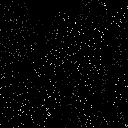## STDIMAGE

 Computes a pixel-by-pixel standard deviation or variance image from multiple input images.

 USAGE: stdimage [-m mode] [-c] infile1 ... infileN outfile USAGE: stdimage [-h or -help] -m .... mode ...... output statistic; mode=s or std (for standard deviation) ................... or mode=v or var (for variance) -c ................ apply contrast stretch to output Note: all images must be the same size PURPOSE: To compute a pixel-by-pixel standard deviation or variance image from multiple input images. DESCRIPTION: STDIMAGE computes a pixel-by-pixel standard deviation or variance image from multiple input images. ARGUMENTS: -m mode ... MODE statistical measure generated in the output image. Choices are either mode=s or std (for standard deviation) or mode=v or var (for variance). The default=std. -c ... Apply contrast stretch to output. CAVEAT: No guarantee that this script will work on all platforms, nor that trapping of inconsistent parameters is complete and foolproof. Use At Your Own Risk.

### EXAMPLES

 Standard Deviation Of Image Vs. Image With Noise Image Noisy Image Arguments: -m std -cStandard Deviation Of Three Completely Different Images Image 1 Image 2 Image 3 Arguments: -m std -cWhat the script does is as follows: Merges all input images into a multi-frame miff image, \$tmp0, from an array of files, \${fileArray[*]} converted to a list, \$infilelist Separates frames and computes standard deviation among all image for each pixel This is equivalent to the following IM commands for the case of standard deviation with contrast stretch ww=`convert "\${fileArray}" -ping -format "%w" info:` hh=`convert "\${fileArray}" -ping -format "%h" info:` convert -size \${ww}x\${hh} xc: \$tmp0 for img in \$infilelist; do convert \$tmp0 \$img \$tmp0 done # note -gamma 0.5 is equivalent to squaring and -gamma 2 is equivalent to square root convert \( \$tmp0 -coalesce -delete 0 -gamma 0.5 -average \) \ \( \$tmp0 -coalesce -delete 0 -average -gamma 0.5 \) \ +swap -compose minus -composite -gamma 2 -contrast-stretch 0 \$outfile